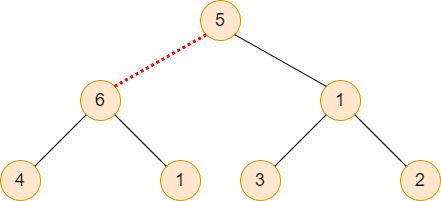# Break The Tree

Posted: 24 Mar, 2021
Difficulty: Moderate

## PROBLEM STATEMENT

#### You are given a binary tree whose each node is associated with some integer value. You have to break this tree into two parts by removing exactly one edge. You also have to maximise the product of the sum of the integers associated with each node in both parts.

##### For Example:``````Consider the above example, if we break the tree by removing the edge between nodes that has integers 5 and 6 then we get the maximum product of the sum of integers on nodes i.e. ( (6 + 4 + 1) * (5 + 1 + 3 + 2) ) = 121.
``````
##### Note:
``````The answer may be very large so, you have to take the modulo with 10^9 + 7.
``````
##### Input Format :
``````The first line contains an integer 'T' which denotes the number of test cases or queries to be run. Then the test cases are as follows.

The first line of each test case contains elements of the tree in the level order form. The line consists of values of nodes separated by a single space. In case a node is null, we take -1 in its place.

For example, the input for the tree depicted in the below image would be :
````````````1
2 3
4 -1 5 6
-1 7 -1 -1 -1 -1
-1 -1
``````

#### Explanation :

``````Level 1 :
The root node of the tree is 1

Level 2 :
Left child of 1 = 2
Right child of 1 = 3

Level 3 :
Left child of 2 = 4
Right child of 2 = null (-1)
Left child of 3 = 5
Right child of 3 = 6

Level 4 :
Left child of 4 = null (-1)
Right child of 4 = 7
Left child of 5 = null (-1)
Right child of 5 = null (-1)
Left child of 6 = null (-1)
Right child of 6 = null (-1)

Level 5 :
Left child of 7 = null (-1)
Right child of 7 = null (-1)

The first not-null node(of the previous level) is treated as the parent of the first two nodes of the current level. The second not-null node (of the previous level) is treated as the parent node for the next two nodes of the current level and so on.
The input ends when all nodes at the last level are null(-1).
``````
##### Note :
``````The above format was just to provide clarity on how the input is formed for a given tree.
The sequence will be put together in a single line separated by a single space. Hence, for the above-depicted tree, the input will be given as:

1 2 3 4 -1 5 6 -1 7 -1 -1 -1 -1 -1 -1
``````
##### Output Format:
``````For each test case, print the maximum product of the sum of integers associated with each node in both the broken parts of the tree.

Output for every test case will be printed in a separate line.
``````
##### Note:
``````You don’t need to print anything; It has already been taken care of.
``````
##### Constraints:
``````1 <= T <= 10
2 <= N <= 100000
1 <= data <= 10^5

Time limit: 1 sec
``````Approach 1

The basic idea is to first calculate the sum of integers associated with each node in the given binary tree and then perform postorder traversal and store the maximum possible answer that can be achieved if we remove this parent from the rest of the tree.

We are here using two helper functions named “calSum” and “postTraversal”.

The “calSum” function is recursive in nature and is used to calculate the sum of integers associated with each node.

``long calSum(TreeNode<int> *root)``

The “postTraversal” function is also recursive in nature and is used to perform the postorder traversal on the given binary tree and it also computes the answer at each recursive call. It returns the maximum possible product of the sum of the integers associated with each node in both parts after removing exactly one edge.

``long postTraversal(TreeNode<int> *root, long totSum, long ans)``

Where “root” is the root node of the given binary tree, “totSum” is the total sum of integers associated with each node, and “ans” is the maximum product of the sum of the integers associated with each node in both parts.

The steps are as follows:

1. In the “calSum” function
• Check is “root” is Null then return 0.
• Computes the sum for left subtree and right subtree.
• Return the total sum of left, right, and the value of “root”.
2. In the “postTraversal” function
• Check is “root” is Null then return 0.
• Computes the sum for left subtree and right subtree.
• Store the total sum of left, right, and the value of “root” in a variable named “curSum”.
• Store the maximum between “ans” and ( “curSum” * (“totSum” - “curSum”) ) in “ans”.
• Return “curSum”.
3. In the given function
• Create two variables “sum” and “ans” to store the total sum and the answer. Initialize both of them with 0.
• Now, call the “calSum” function first and then “postTraversal” function.
• Return “ans” after taking modulo with 10^9 + 7.